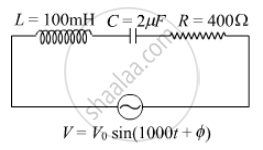# Find the value of the phase difference between the current and the voltage in the series LCR circuit shown below. Which one leads in phase : current or voltage ? - Physics

(i) Find the value of the phase difference between the current and the voltage in the series LCR circuit shown below. Which one leads in phase : current or voltage ?

(ii) Without making any other change, find the value of the additional capacitor C1, to be connected in parallel with the capacitor C, in order to make the power factor of the circuit unity.#### Solution

(i) Given V = V0sin(1000t + ϕ)
ω = 1000 s-1

Given,
L = 100 mH
​C = 2 μF
R = 400 Ω

Phase difference ϕ= tan-1((XL−XC)/R)

XL = ωL = 1000 × 100 × 10​-3 = 100 Ω
X_C=1/(omegaC)=1/(1000xx2xx10^-6)=500Omega

ϕ= tan^-1((100−500)/400)= tan^-1(-1)

ϕ = -450 and the current is leading the voltage.

(ii)
For power factor to be unity, R = Z

or XL = XC
ω^2 =1/(LC) (C = resultant capacitance)
10^6 = 1/(100xx10^-3xxC')

⇒C' = 10-5 F

For two capacitance in parallel, resultant capacitance C'= C + C1

10-5 = 0.2 x 10-5 + C1
⇒C1 = 8 μF

Is there an error in this question or solution?
2016-2017 (March) Delhi Set 1

Share